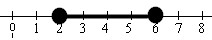Linear Inequalities (single variable)

Example 1:    Solve the inequality: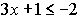• Enter each side of the inequality into Y1 and Y2

• Enter the inequality statement into Y3: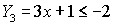(The inequality symbols are under the TEST Menu - 2nd MATH.)
This step could also be entered as Y1 < Y2.

• Graph.  Use the intersect option (2nd Trace #5 Intersect) to find the exact point where the inequality answer begins.

• Do a Boolean Check:  Where the inequality is true, the y-values on the graph will be a 1.

• If you look at the table, 0's will be listed where the inequality is false and 1's will be listed where the inequality is true.  (arrow to the right to see additional tables)

• Remember that the calculator cannot draw an open or closed circle on the intervals. You will have to determine which circle is needed based upon whether the inequality includes "equal to".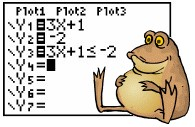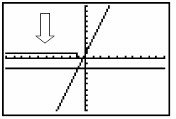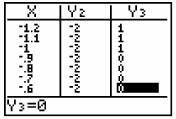If you turn off the axes (FORMAT - 2nd ZOOM), you will be able to see the graphing of the 0's and 1's more clearly.  Notice that the small vertical segment connecting the 0's to the 1's is simply the calculator being set in "connected" mode.  Change to "dot" mode to eliminate this segment.
Example 2:     Solve the inequality: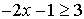• Enter the inequality into Y1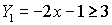• Graph
• If you enter the inequality directly into Y1, you will see only the Boolean graph.  It may be necessary to look carefully in your table to find the exact point where the inequality answer begins.
• Such answers can be found easily if the answers are integer values.  Otherwise, you will want to use the intersect option mentioned above to find an exact answer.
• In this problem, the calculator automatically took care of reversing the direction of the inequality when solving.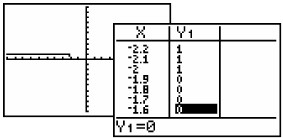Example 3:    Graph the solution set for   2x - 4 < 8   and   x + 5 > 7

Algebraic solution:  Graphical solution:
 Solve each equation separately.  The answer will be points that make BOTH sets true. Both inequalities are entered into Y1 with the use of "and".   Find "and" by using the CATALOG (2nd 0) or  TEST (2nd MATH)→ LOGIC, #1 and.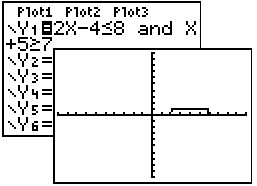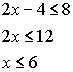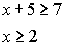Answer:  2 < x < 6